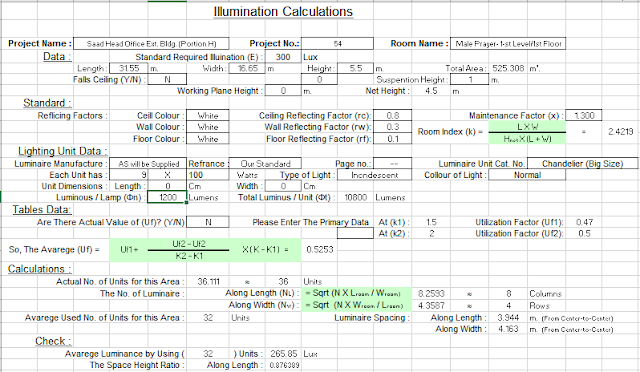Free excel sheet for illumination and lighting calculation for building. This spreadsheet calculates the required illumination for each room in a building.Lighting Calculations Excel Sheet

## Factors affected on illuminance calculations

### 1. Room Reflectance

The room is considered to consist of three main surfaces:
(a) the ceiling cavity,
(b) the walls, and
(c) the floor cavity (or the horizontal working plane).

The effective reflectances of the above three surfaces affect the  quantity of reflected light received by the working plane

### 2. Luminaire maintenance factor (LMF)

is the proportion of the initial light output from a luminaire after a set time to the initial light output from a lamp after a set time.

Three factors must be considered in its determination:
(a) the type of luminaire,
(b) atmospheric conditions, and
(c) maintenance interval.

### 3. Room surface maintenance factor (RSMF)

is the proportion of the illuminance provided by a lighting installation in a room after a set time compared with that occurred when the room was clean

### 4.Utilization factor (UF)

is the proportion of the luminous flux emitted by the lamps which reaches the working plane.
Factors that affect the value of UF are as follows:
(a) light output ratio of luminaire
(b) flux distribution of luminaire
(c) room proportions
(d) room reflectance
(e) spacing/mounting height ratio